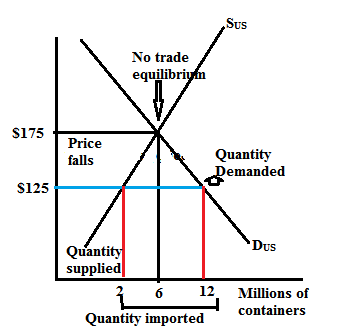# Quiz 15: International Trade Policy

Business

(a) Without International trade the price of a container will be \$175 and quantity demanded will be equal to quantity supplied that is 6 millions of containers per year. (b) Wholesalers can buy roses at auction from Holland fro \$125 which is less than the domestic prices. Thus rest of the world has comparative advantage in producing roses except US.

If the US wholesalers buy roses at the lowest possible prices that is at \$125 so, at this price quantity demanded is 12 million while quantity supplied is only 2 millions. Hence, import would be of 10 millions of containers per year.

The below graph represents the equilibrium prices without international trade and with international trade.The demand curve D US and the supply curve S US show the demand and supply in the US domestic market only. The demand curve tells us the quantity of roses that Americans are willing to buy at various prices. The supply curve tells us the quantity of roses that US makers are willing to sell at various prices. Without International trade the price of a container will be \$175 and quantity demanded will be equal to quantity supplied that is 6 millions of containers per year. If the US wholesalers buy roses at the lowest possible prices that is at \$125 so, at this price quantity demanded is 12 million while quantity supplied is only 2 millions. Hence, import would be of 10 millions of containers per year

There is no answer for this question

There is no answer for this question

There is no answer for this question